## The breakdown of a sample of a chemical compound is represented by the function p(t) = 500 (0.25)^t, where p(t) represents the number of mil

Question

The breakdown of a sample of a chemical compound is represented by the function p(t) = 500 (0.25)^t, where p(t) represents the number of milligrams of the substance and t represents the time. In years. In the function p(t), explain what 0.25 and 500 represent.​

in progress 0
2 weeks 2021-09-05T14:21:43+00:00 1 Answers 0 views 0

1. Given:

The function is: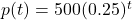Where p(t) represents the number of milligrams of the substance and t represents the time.

To find:

The correct explanation for the number 0.25 and 500 in the given function.

Solution:

The general exponential function is: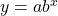…(i)

Where, a is the initial value and b is the growth/decay factor. If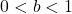, then decay factor and if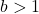, then growth factor.

We have,…(ii)

On comparing (i) and (ii), we get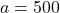, it means initial there are 500 milligrams of the substance.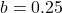, this value is less than 1, it means the substance is decreasing by a factor of 0.25.

Therefore, 0.25 means the substance is decreasing by a factor of 0.25 and 500 means the initial value of substance is 500 milligram.